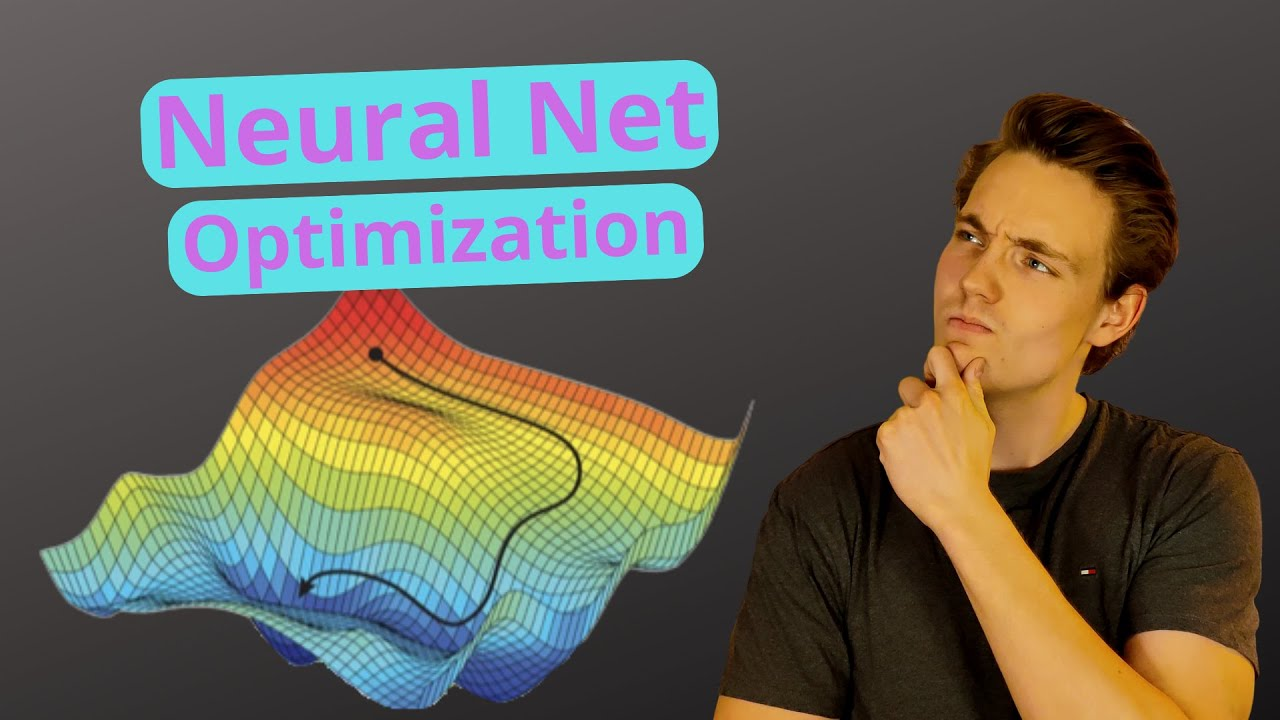# Optimizers, Loss Functions and Learning Rate in Neural Networks with Keras and TensorFlowIn this Neural Networks Tutorial, we will talk about Optimizers, Loss Function, and Learning rate in Neural Networks. I will explain what an optimizer is and how it is used in the training process of a neural network.

In this Neural Networks Tutorial, we will talk about Optimizers, Loss Function, and Learning rate in Neural Networks. I will explain what an optimizer is and how it is used in the training process of a neural network. We are going to see some different types of optimizers and loss functions. The objective of a neural network during training is to minimize the loss function with an optimizer with a chosen learning rate. At the end of the video, we are going to compile the neural network that we have created with the chosen optimizer and loss function. Throughout this tutorial, we are going to cover everything about the basics and fundamentals of neural networks. How they work, how you can create one yourself, and how you can train it to make actual predictions on data the network has not seen before.

Code examples and images from this tutorial will be available on my GitHub: https://github.com/niconielsen32​

## Keras vs. Tensorflow - Difference Between Tensorflow and Keras

Keras vs Tensorflow - Learn the differences between Keras and Tensorflow on basis of Ease to use, Fast development,Functionality,flexibility,Performance etc

## TensorFlow And Keras Tutorial | Deep Learning With TensorFlow & Keras | Deep Learning

This video on TensorFlow and Keras tutorial will help you understand Deep Learning frameworks, what is TensorFlow, TensorFlow features and applications, how TensorFlow works, TensorFlow 1.0 vs TensorFlow 2.0, TensorFlow architecture with a demo. Then we will move into understanding what is Keras, models offered in Keras, what are neural networks and they work.

## Pytorch vs Tensorflow vs Keras | Deep Learning Tutorial (Tensorflow, Keras & Python)

We will go over what is the difference between pytorch, tensorflow and keras in this video. Pytorch and Tensorflow are two most popular deep learning frameworks. Pytorch is by facebook and Tensorflow is by Google. Keras is not a full fledge deep learning framework, it is just a wrapper around Tensorflow that provides some convenient APIs.

## Keras Tutorial - Ultimate Guide to Deep Learning - DataFlair

Keras Tutorial - Learn Keras Introduction, installation, Keras Features, Applications of Keras, Keras Layers, Keras models and keras visualize training.

## Keras Tutorial For Beginners | What is Keras | Keras Sequential Model | Keras Training

🔵 Intellipaat Artificial Intelligence Master's Course: https://intellipaat.com/artificial-intelligence-masters-training-course/🔵 In this video on Keras, ...